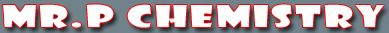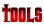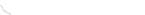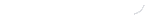activity seriesbond energybond lengthscalculatorcommon namesconversion factors: energyconversion factors: lengthconversion factors: massconversion factors: pressureconversion factors: temp.conversion factors: volumecovalent prefixesdensity solverdiatomic elementse-config. chartelement listgas law formulasKa's of polyprotic acidsKa's of weak acidsKb's of weak basesmetric conversion chartmole conversion chartmolecular geometriesorganic prefixesperiodic tableperiodic table (flash)pH/pOH converterpolyatomic ionspressure converterSI unitssolubility chartsolubility of salts rulessolubility product constantsstoichiometry charttemp. conversiontemp. formulasthermodynamic datavapor pressure of water
Compounds in Crust of Earth

4
 The 10 Most Abundant Compounds in the Earth's Crust Compound Formula Abundance by weight silicon dioxide SiO2 42.86% magnesium oxide MgO 35.07% iron(II) oxide FeO 8.97% aluminum oxide Al2O3 6.99% calcium oxide CaO 4.37% sodium oxide Na2O 0.45% iron(III) oxide Fe2O3 0.36% titanium dioxide TiO2 0.33% chromium(III) oxide Cr2O3 0.18% manganese(IV) oxide MnO2 0.14% Total 99.72%

 You may also find these related tutorials helpfulCompounds in Crust of EarthDensityElements in Crust of EarthHow To Round NumbersMetric ConversionScientific NotationSI UnitsSignificant Figure CalculationsSignificant Figures